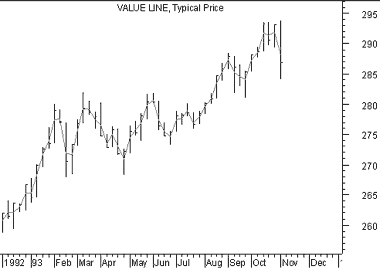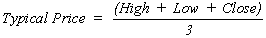# Technical Analysis from A to Z

by Steven B. Achelis

TYPICAL PRICE

Overview

The Typical Price indicator is simply an average of each day's price. The Median Price and Weighted Close are similar indicators.

Interpretation

The Typical Price indicator provides a simple, single-line plot of the day's average price. Some investors use the Typical Price rather than the closing price when creating moving average penetration systems.

The Typical Price is a building block of the Money Flow Index.

Example

The following chart shows the Typical Price indicator on top of Value Line's bar chart.Calculation

The Typical Price indicator is calculated by adding the high, low, and closing prices together, and then dividing by three. The result is the average, or typical price.This online edition of Technical Analysis from A to Z is reproduced here with permission from the author and publisher.